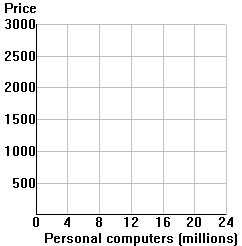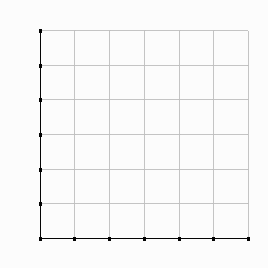Market equilibrium worksheet

1.

In the diagram to the right, plot the following hypothetical supply and demand information for personal computers (PCs):Quantity Demanded (Qd - millions) Price (\$) Quantity Supplied (Qs - millions) 2 \$3,000 17 4 2,500 16 7 2,000 14 11 1,500 11 16 1,000 7 22 500 2

 2. The equilibrium price "clears the market," in that quantity demanded equals quantity supplied. The equilibrium price = ______

3.At a price of \$2,500... At a price of \$1,000... (1) Is there excess demand or excess supply? (2) How many million units? (3) Are PC inventories rising or falling? (4) Is the incentive to raise or lower price?

4.

Indicate whether equilibrium price (P) and quantity (Q) will rise (+) or fall (-) if ...
 Price Quantity (1) Demand shifts right (2) Demand shifts left (3) Supply shifts right (4) Supply shifts left